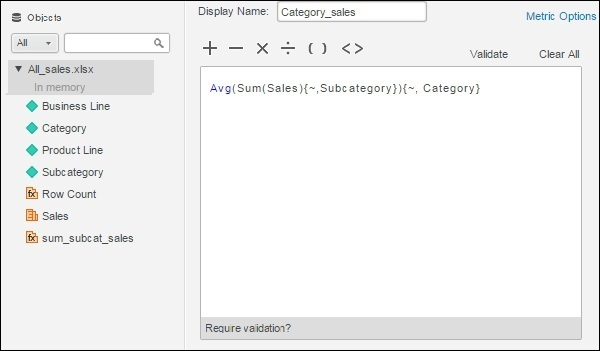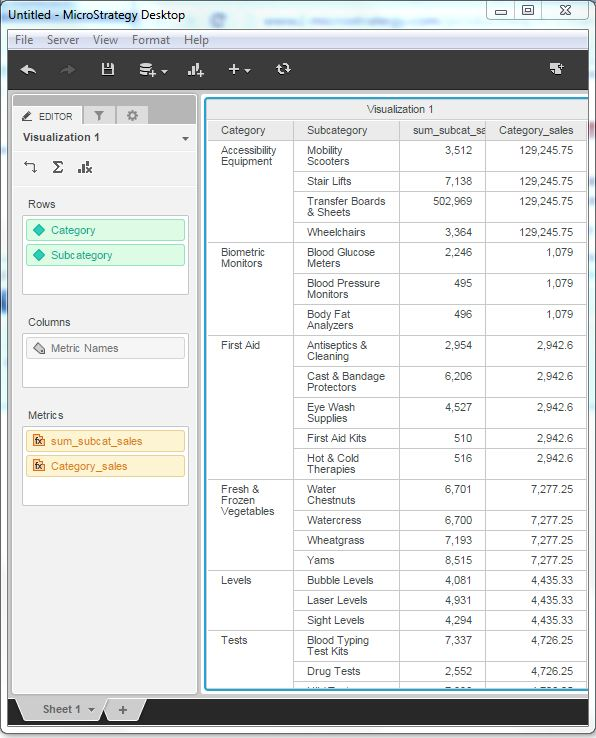# MicroStrategy - Nested Metrics

Nested Metric in MicroStrategy are the calculations in which one aggregation function is enclosed inside another. They are useful when in the data warehouse design, we do not have data stored at the required level of granularity. In such case, we create an inner formula and an outer formula. Combining them creates the nested metric.

## Example

In this example, we aim to find the average sales for each sub-category as compared to the total sales under each category.

## Step 1

Create a report with Category and sub-category as its two columns. Next, right-click anywhere under the data source tab and near any of the measure fields. A pop-up appears which shows the create metric option. We create the first metric with the following formula and name it as sum_subcat_sales.## Step 2

Next, we create another metric with the name Category_sales. In it, we write the inner formula for the sum of sales for each category and the outer formula giving average sales for each category, corresponding to the sub-category.## Step 3

Finally, drag both the newly created metrics to the report to see the result.